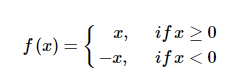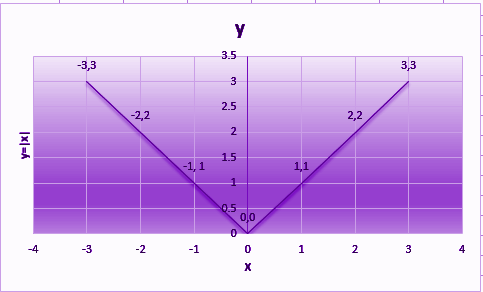# Modulus Function

A modulus function is a function which gives the absolute value of a number or variable. It produces the magnitude of the number of variables. It is also termed as an absolute value function. The outcome of this function is always positive, no matter what input has been given to the function. It is represented as y = |x|. The plotting of such graphs is also an easy method where the domain will be all values of input say x (all real numbers) and range will be all values of function (y = f(x) = all positive real numbers and 0). This topic is covered under relation and functions of Class 11 Maths.

## Modulus Function Definition

A relation ‘f’ is called a function, if each element of a non-empty set X, has only one image or range to a non-empty set Y. The modulus function f(x) of x is defined as;

f(x) = |x|

Or

y = |x|

Where f:R→R and x ∈ R

And |x| states modulus or mod of x.

If x is positive then the output of the function f(x) will be x only. But if x is negative, then the output of x will be the magnitude of x. Hence, we can redefine the modulus function as:According to the above statement, if the value of x is greater or equal to 0, then the modulus function takes the actual value. But if the value of x is less than 0, then the function takes minus of the actual values. Let us see some examples to understand it:

If x = -5, then y = f(x) = – (-5) = 5, since x is less than zero

If x = 10, then y = f(x) = 10, since x is greater than zero

If x = 0, then y = f(x) = 0, since x is equal to zero

### Modulus Function Graph

Now let us plot a graph for modulus function. Let x be variable whose values lies from -3 to 3, which is the domain of the graph. So, the range of function f(x) should be always positive for all values of x, as per the definition. Suppose x-axis shows the value of variable x and the y-axis shows the value of function y, then we can plot the graph as per the given values in the table here.

 x<0 x≥0 x -3 -2 -1 0 1 2 3 y 3 2 1 0 1 2 3

As per the given values above, the graph of modulus function is plotted here.You can see from the above graph, the values of modulus function stay positive for all the values of x, such as;

• When x = -3 then y = |-3| = 3
• When x = -2 then y = |-2| = 2
• When x = -1 then y = |-1| = 0
• When x = 0 then y = |0| = 0
• When x = 1 then y = |1| = 1
• When x = 2 then y = |2| = 2
• When x = 3 then y = |3| = 3

## Video Lesson

### Graph of Modulus Function### Modulus Function Questions

Since you have learned all the details about modulus function and how to plot the graph for such functions, practice some questions given below based on it.

1. If y = 2x +1 is a modulus function, plot the graph for it
2. If f(x) = tan x is a modulus function, show the function graphically
3. If f(x) = x2 + 2 is modulus, then plot the graph for this function